# A new AC – AC converter with buck and boost options

A new AC – AC converter with buck and boost options

Al-Habeeb College of Engineering and Technology, Chevella, RR Dt., India

Electrical Engineering Department, King Khalid University, Kingdom of Saudi Arabia

Engineering Department, Shinas College of Technology, Al Aqar, Sultanate of Oman

Corresponding Author Email:
Karim.02214@gmail.com
Page:
295-308
|
DOI:
https://doi.org/10.3166/EJEE.20.295-308
|
Accepted:
|
Published:
30 June 2018
| Citation

OPEN ACCESS

Abstract:

This paper proposes a novel AC-AC converter capable of operating in non-inverting and inverting buck boost modes. The projected AC-AC converter uses six IGBTs with reverse blocking capability, two capacitors and one inductor. The main advantage of this AC-AC converter is that it is free from shoot through problem even when all the six IGBTs are in ON condition. Hence dead time between two pulse width modulation signals is not necessary, which results in load voltage waveform with very less Total Harmonic Distortion (THD). Furthermore elimination of dead time, eradicates the commutation problem even in the absence of hefty snubbers or with soft commutation methods. The switching losses of the proposed converter are very less since only two devices are switched at high frequency that too only in a half cycle. The remaining switches are switched at fundamental frequency, which in turn increases the efficiency of the converter. The proposed converter is a suitable candidature in dynamic voltage restorer and single phase solid state transformer applications.

Keywords:

commutation, AC-AC converter, buck-boost modes, inverting and non-inverting

1. Introduction

Conventional thyristor-based ac-ac converters are used in industries for the past few decades, which uses either integral cycle control or phase angle control to obtain the required output voltage across the loads. But the major drawbacks of the conventional ac voltage controllers are large THD in the input current, less efficiency and low input power factor, which restricts their applications as described by Nguyen et al. (2010). In certain applications where both variable voltage and frequency is demanded, indirect ac-ac converters (i.e.AC-DC-AC Converters) with DC link or direct ac-ac converters (i.e. Matrix converters) are used because of their improved efficiency and power factor as shown in Liu et al. (2009). In addition, their filter size requirements are also less when compared to ac voltage controller. On the other hand, applications which demands only voltage control, Pulse Width Modulation (PWM) ac voltage regulators are used because of their compact size and low cost as described by Jacobina et al. (2006). These PWM ac voltage regulators shown in Alemi et al. (2015), Ecklebe et al. (2009) and Cardenas et al. (2012) are modified versions of the DC-DC converters, where all the unidirectional devices are changed into bi-directional switches. On the contrary, all they type of PWM ac voltage regulators have their own boundaries; for example, boost type PWM ac voltage regulator can only boost up the input voltage whereas on other hand the buck type PWM ac voltage regulator can only step down the input voltage. There are other versions like buck boost and cuk regulators where it is possible to increase the load voltage above the level of input voltage and also decrease it below the input voltage. But the main restriction of the buck-boost and cuk type topology is that the load voltage phase is reversed with respect to the input voltage as demonstrated in Metidji et al. (2013), Xia et al. (2014) and Ge et al. (2014). Additionally, in both these topologies the voltage stresses across the switches are much higher which limit their applications.

Furthermore, in buck boost topology, both the input and output current are discontinuous in nature which restricts its usage. Where as in the case of cuk regulator the input and output currents are continuous in nature, because of its additional passive components, but this increases its cost and size of the converter. Quasi Z source (qZS) ac-ac converters are also proposed in the literature, which operates in the buck and buck boost modes. During the operation of qZS in buck mode the phase of the load voltage reverses with respect to the input voltage, further during the shoot through state of the switches enormous amount of current flow through the device which limits their use. Flying capacitor based buck type multilevel inverter is proposed in Peng et al. (2003), Sarnago et al. (2014) and Kim et al. (1998), which improves the load voltage profile in terms of its total harmonic distortion (THD) and also reduces the voltage stress on the switches. But in this topology in order to maintain flying capacitor voltages at a constant value an RLC network is added which increases its size and complexity as in Vicuna et al. (2009), Moghe et al. (2015) and Li and Zhong (2012).

All these topologies have commutation problem. Ideally complementary devices do not have any overlap or dead-time, but practically there is a very small overlap between the gating signals of the complementary switches as in Fang et al. (2005), Nguyen et al. (2012) and Banaei et al. (2015). During this overlap, either a short circuit of voltage source occurs or two capacitors of different voltages come in parallel to each other. This results in large current spikes across the devices which lead to damage them. To evade this damage to the switching devices a small dead time is added to the gating signals to avoid overlap, further hefty and lossy RC snubbers are used to protect the devices from voltage or current spikes as in Tang et al. (2007) and He, Duan and Peng. (2013). Gagliano and Nocera (2017). proposes the effective way for electric energy storage in residential applications.

To solve the above drawbacks of existing and conventional ac-ac converters, a direct ac-ac converter is proposed which combines the functions of inverting buck-boost converters and non-inverting buck-boost converters in a single topology.

2. Proposed convertor topology

## 1.png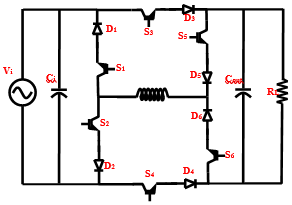Figure 1. Proposed AC-AC converter

Figure 1 shows the proposed AC-AC converter topology which has six power electronic devices, six diodes, two filter capacitors and one inductor. The proposed AC-AC converter topologies is capable of operating as inverting buck and boost converter as well as non-inverting buck and boost converter. The main application of the proposed AC-AC converter topology is that it can be used as Dynamic Voltage Restorer (DVR), where the non-inverting buck and boost converters can be operated to compensate voltage dips and the inverting buck and boost converters can be operated to compensate the voltage swells. Further the main advantage of the proposed converter topology is that it is free from commutation problem.

2.1. Study of Commutation Strategy in the projected converter

In the proposed converter topology switches S1 and S2 are complementary to each other. Similarly switches S3 and S4, S5 and S6 are complementary to each other. Generally if there is no dead band between complementary switches which may lead to short circuit at the input side or at the output side based on the operation. But here in the projected converter topology, when S1& S2 are turned on at the same instant of time as shown in figure 2(a), diode D1 will not allow the current to flow through the devices S1& S2 which prevents the problem of short circuit the input capacitance. Hence switches S1 and S2 are commutation free switches. Further as shown in figure 2(b) the switches S3 and S4 can be turned on at the same instant of time, during this mode of operation diode D4 prevents current flowing through the devices S3 and S4, which eliminates the problem of short circuit the output filter capacitance. Similarly as shown in figure 2(c) when switches S5 and S6 are turned on at the same instant of time, diode D6 eliminates the short circuit problem of the load side capacitance. Hence from figures 2(a), 2(b) and 2(c) the proposed AC-AC converter topology is free from the commutation problem.

## 2a.png(a) State 1

## 2b.png(b) State 2

## 2c.png(c) State 3

Figure 2. Possible shoot through

2.2. Non-inverting mode of operation

The proposed inverter can operate in both non-inverting and inverting modes of operation. Further in each of these modes the converter is capable of operating as buck and boost converter. In this section the operation of the proposed converter in non-inverting mode is dealt in detail.

2.2.1. Proposed topology operating as buck converter

The proposed topology has four modes of operation as indicated in Table (1). Figure 3(a) shows the proposed topology operating as buck converter in non-inverting mode when the input voltage is greater than 0 during duty cycle interval. Similarly figure 3(b) shows the operation of the proposed topology operating as buck converter in non-inverting mode when the input voltage is greater than 0 during OFF duty cycle interval. Furthermore figure 4(a) and 4(b) shows the operation of the proposed converter as buck converter in non-inverting mode when the input voltage is greater than 0 during duty cycle and OFF duty cycle interval respectively. Figure 7(a) shows the key operating waveforms of the proposed converter topology in buck mode.

## 3a.png(a) duty cycle interval

(i.e. during DT interval)

## 3b.png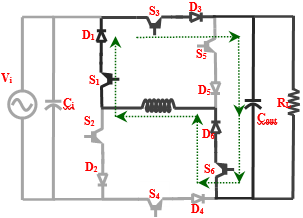(b) OFF duty cycle interval

(i.e.  during (1-D) T interval)

Figure 3. Buck operation - non-inverting mode when input voltage > 0 during

## 4a.png(a) duty cycle interval

(i.e. during DT interval)

## 4b.png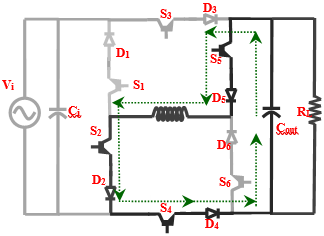(b) OFF duty cycle interval

(i.e. during (1-D) T interval)

Figure 4. Buck operation – non-inverting mode when input voltage < 0 during

For Vin<0, switches S2, S4, S5 are always turn on while switches S3, S6 are always turn off, and S1becomes high frequency switch. The operation for Vin<0 is same as explained for Vin>0, with only difference is that now the switch S1 performs same as S2 (for Vin>0), and vice versa. The equivalent circuits during this negative half-cycle are shown in Figure. 4(a) and (b).

Table 1. Switching table for non-inverting buck mode

 Input Voltage S1 S2 S3 S4 S5 S6 Reference Vin > 0 OFF ON ON OFF OFF ON Figure 3(a) ON OFF ON OFF OFF ON Figure 3(b) Vin < 0 ON OFF OFF ON ON OFF Figure 4(a) OFF ON OFF ON ON OFF Figure 4(b)

2.2.2. Proposed topology operating as boost converter

The switching table of proposed topology operating as boost converter in non-inverting mode is given in Table (2). Figure 5(a) shows the operation of the proposed topology operating as a boost converter in non-inverting mode. From figure 5(a) switches S2, S3 and S5 are in ON condition when the input voltage Vin is greater than 0 (i.e. during the positive half cycle of the input voltage). Immediately after this operation switch S5 is turned off and S6 is turned on as shown in Figure 5(b). The switching sequence of the proposed converter during non-inverting boost mode operation and key waveforms are shown in Figure. 7(a). For Vin>0, switches S2, S3, S6 are always turn on and S1, S4 are always turn off, while switch S5 is switched at high frequency.

## 5a.png(a) duty cycle interval

(i.e. during DT interval)

## 5b.png(b) OFF duty cycle interval

(i.e. during (1-D) T interval)

Figure 5. Boost operation – non-inverting mode when input voltage > 0 during

Figure 5 and figure 6 shows the equivalent circuits of the proposed converter for Vin>0. The circuit shown in Figure. 5(a) is during DT interval in which switch S5 is turned on and the input energy is stored in inductor L. The switch S6 is also turned on; however, its external series diode becomes reverse biased because of inverse output voltage V0across it. Therefore, no current flows through switch S6 during this interval, as shown in Figure. 6(b).

Table 2. Switching table for Non-Inverting buck mode

 Input Voltage S1 S2 S3 S4 S5 S6 Reference Vin > 0 OFF ON ON OFF ON OFF Figure 5(a) OFF ON ON OFF OFF ON Figure 5(b) Vin < 0 ON OFF OFF ON OFF ON Figure 6(a) ON OFF OFF ON ON OFF Figure 6(b)

## 6a.png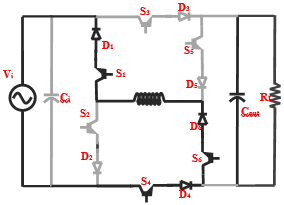(a) duty cycle interval

(i.e. during DT interval)

## 6b.png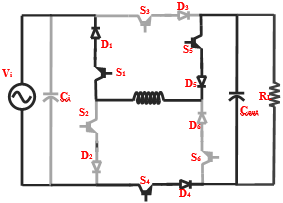(b) OFF duty cycle interval

(i.e. during (1-D)T interval)

Figure 6. Boost operation - non-inverting mode when input voltage < 0 during

## 7a.png(a) Buck Mode

## 7b.png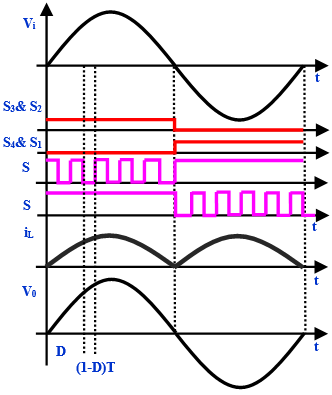(b) Boost Mode

Figure 7. Non inverting modes of operation key waveforms

For Vin<0, switches S1, S4, S5 are always turn on while switches S2, S3 are always turn off, and S6 becomes high frequency switch. The operation for Vin<0 is the same as explained for Vin>0, with only difference is that now the operation of switch S6 is same as that of S5 (for Vin>0), and vice versa. The equivalent circuits during this half-cycle are shown in Figure. 7(a) and (b).

2.3. Inverting mode of operation

The switching sequence of the proposed converter during inverting buck–boost mode operation and key waveforms are shown in Figure. 8. For Vin> 0, switches S2, S4, S5 are always turn on and S1, S6 are always turn off, while switch S3 is switched at high frequency. Figure 9 shows the equivalent circuits of the proposed converter for Vin> 0. The circuit shown in Figure 9(a) is during DT interval in which switch S3 is turned on and the input energy is stored in inductor L. The switch S4 is also turned on; however, its external series diode becomes reverse biased because of inverse voltage (Vin + Vo) across it. Therefore, no current flows through switch S4 during this interval, as shown in Figure 9(a).

## 8.pngFigure 8. Inverting mode of operation key waveforms in buck-boost mode

## 9.png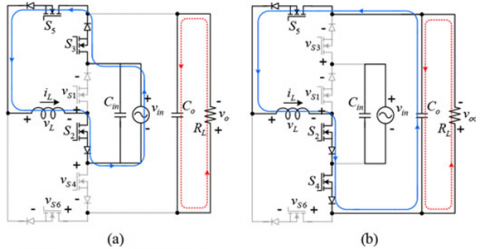Figure 9. Boost operation - inverting mode when Vin> 0 (a) during DT interval (b) (1-D) T interval

For Vin<0, switches S1, S3, S6 are always turn on while switches S2, S5 are always turn off, and S4 becomes high frequency switch. The operation for Vin<0 is the same as explained for vin >0, with only difference is that now the switch S4 acts the same as S3 (for Vin>0), and vice versa. The equivalent circuits during this half-cycle are shown in Figure. 10(a) and (b). The details of switching strategy are shown in table (3).

## 10.png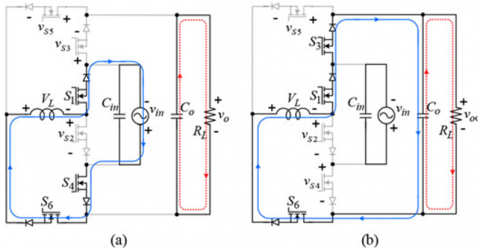Figure 10. Boost operation - inverting mode when Vin< 0 (a) during DT interval (b) (1-D) T interval

3. Simulation results

To validate the proposed AC-AC converter, a simulation model is developed in MATLAB/SIMULINK environment and the simulation results are presented in this section. The various simulation parameters used in this study are shown in Table (3). The switching signals of all the switches in non-inverting mode for Buck action is shown in Figure 11. From Figure 11 it can be seen that two switches operate at fundamental frequency and remaining four switches operate at high frequency.

## 11.pngFigure 11. Switching signals of Non-Inverting mode buck AC-AC converter

Table 3. Simulation parameters

 Switching Frequency 25kHz Peak input Voltage 135V Frequency 50Hz Load Resistance 100 ohms Input Capacitance 1.5 micro farads Output Capacitance 4.5 micro farads Solver type Ode23s (stiff/Mod. Rosen Brock) Max step size 1e-6 Min step size 1e-7

Figure 12 shown the input voltage, load voltage and load current of the proposed ac-ac converter operating in non-inverting mode (in buck mode) for duty cycle of 0.4. It is evident from Figure 12 that the load voltage is below 100-volt peak whereas the input voltage is 135V peak. It should be noted that when the duty cycle is between 0 to 0.5 it operates in buck mode, whereas for the proposed converter to be operated in boost mode the duty cycle of the converter should be more that 0.5 and less than 1.

## 12.png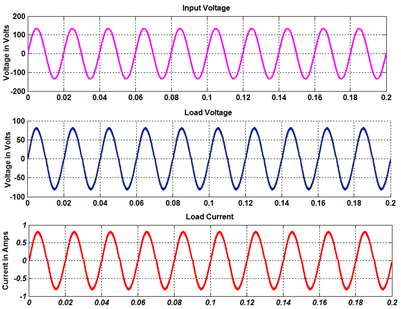Figure 12. Input voltage, load voltage and load current for Non-inverting mode in buck action

Figure 13 shows the input voltage, load voltage and load current waveform of the proposed converter when operating in boost non-inverting mode. The Figure 13 corresponds to the duty cycle of 0.8. It is evident from the Figure 13 that the load voltage is greater than the input voltage i.e. the load voltage has a peak value of 500 volts whereas the peak value of the input voltage is 135 volts. Hence the proposed inverter operates much conveniently in the boost mode. Further the load current is also boosted up as shown in Figure 13.

## 13.png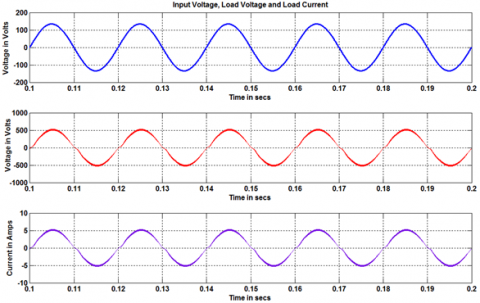Figure 13. Input voltage, load voltage and load current for Non-inverting mode in boost action

Figure 14 shows the switching signals of all the six switches when the converter is operating in inverting buck mode. From Figure 14 it is evident that switches S1, S2, S5 and S6 operate in low fundamental frequency whereas switches S3 and S4 operate at high (i.e. carrier) frequency. The above switching pattern is used to drive the switches for inverting buck mode of operation. Figure 15 shows the input voltage, load voltage and load current of the proposed ac-ac converter when it is operated in inverting buck mode. From Figure 15 it is evident that there is a phase shift of 180 degrees between the input voltage and load voltage, which means that the proposed converter is operating in inverting mode. Further the load voltage peak value is also less than that of the input voltage, which means that the converter is operating in buck mode.

## 14.png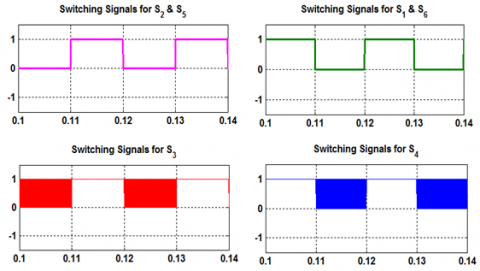Figure 14. Switching Signals for the proposed converter for inverting mode

## 15.png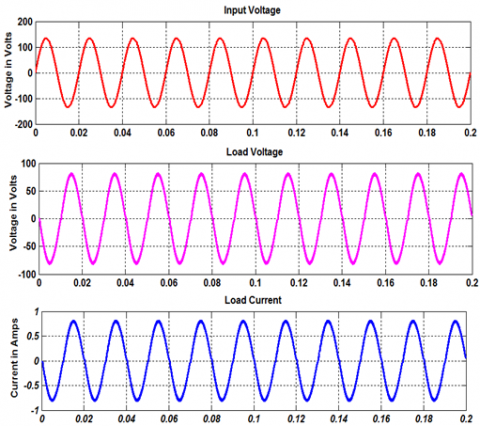Figure 15. Input voltage, load voltage and load current for inverting mode in buck action

Figure 16 shows the input voltage, load voltage and load current waveform of the proposed ac-ac converter operating in inverting boost mode. From Figure 15 it is evident that the load voltage has a phase shift of 180 degrees with respect to the input voltage and also the load voltage magnitude is greater than that of the input voltage magnitude. Thus the proposed ac-ac converter is capable of operating in inverting buck as well as boost modes.

## 16.pngFigure 16. Input voltage, load voltage and load current for inverting mode in boost action

4. Conclusions

In this paper, a single-phase PWM ac–ac converter has been proposed which combined the operation of non-inverting buck and boost converters and inverting buck–boost converter in one topology. The proposed converter uses six unidirectional current flowing bidirectional voltage blocking switches, two capacitors, and one inductor. The proposed converter has no shoot-through problem even when all switches are turned on simultaneously, and therefore, can eliminate PWM dead-times to produce high quality output voltage. Furthermore, the proposed converter can solve commutation problem without using bulky and lossy RC snubber or dedicated soft commutation strategy. In the proposed converter, only two switches are switched at high frequency during each half-cycle, which reduces the switching losses. Moreover, since body diode of switches never conducts the proposed converter can use power IGBTs, which benefits in low switching loss, resistive conduction voltage drops, fast switching speed, etc.

The proposed converter can operate in non-inverting buck and boost modes to replace the inverting buck–boost ac–ac converter with following benefits; decreased switch voltage stresses, inductor current and current ripple, increased reliability because of no commutation problem, and no need of PWM dead-times. In its application as DVR, the inverting buck–boost mode of the proposed converter can also be utilized along with non-inverting buck and boost modes, to compensate both voltage sags and swells. A detailed analysis of the proposed converter has been presented.

References

Ecklebe A., Lindemann A., Schulz S. (2009). Bidirectional switch commutation for a matrix converter supplying a series resonant load. IEEE Trans. Power Electron, Vol. 24, No. 5, pp. 1173-1181. http://doi.org/10.1109/TPEL.2008.2011737

Ge B., Lei Q., Qian W., Peng F. (2014). A family of Z-source matrix converters. IEEE Trans. Ind. Electron, Vol. 59, No. 1, pp. 35-46. http://doi.org/10.1109/TIE.2011.2160512

Metidji B., Taib N., Lotfi B., Toufik R. (2013). Novel single current sensor topology for venturini controlled direct matrix converters. IEEE Trans. Power Electron, Vol. 28, No. 7, pp. 3509-3516. http://doi.org/10.1109/TPEL.2012.2227341

Jacobina C. B., Freitas I., Silva E. D., Lima A. M. N. (2006). Reduced switch count DC-link AC-AC five-leg converter. IEEE Trans. Power Electron, Vol. 21, No. 5, pp. 1301-1310. http://doi.org/10.1109/TPEL.2006.880307

Liu C. W., Wu B., Zargari N. R., Xu D. (2009). A novel three-phase three-leg ac-ac converter using nine IGBTs. IEEE Trans. Power Electron, Vol. 24, No. 5, pp. 1151-1160. http://doi.org/10.1109/TPEL.2008.2004038

Brahim M., Nabil T., Baghli L., Rekioua T. (2014). Novel single current sensor topology for venturini controlled direct matrix converters. IEEE Trans. Power Electron, Vol. 99, No. 1, pp. 5417-5430. http://doi.org/10.1109/TPEL.2012.2227341

Peng F., Chen L. H., Zhang F. (2003). Simple topologies of PWM AC-AC converters. IEEE Power Electron. Lett, Vol. 1, No. 1, pp. 10-13. http://doi.org/10.1109/LPEL.2003.814961·

Gagliano A., Nocera F. (2017). Analysis of the performances of electric energy storage in residential applications. International Journal of Heat and Technology, Vol. 35, Special Issue 1, pp. S41-S48. http://doi.org/10.18280/ijht.35Sp0106

Sarnago H., Lucia O., Mediano A., Burdío J. M. (2014). Direct AC-AC resonant boost converter for efficient domestic induction heating applications. IEEE Trans. Power Electron, Vol. 29, No. 3, pp. 1128-1139. http://doi.org/10.1109/TPEL.2013.2262154

Kim J. H., Min B. D., Kwon B. H., Won S. C. (1998). A PWM buck-boost ac chopper solving the commutation problem. IEEE Ind. Electron. Lett, Vol. 45, No. 5, pp. 832-835. http://doi.org/10.1109/41.720341

Vicuna L. G. G. D., Castilla M., Miret J. (2009). Sliding-mode control for a single-phase ac/ac quantum resonant converter. IEEE Trans. Ind. Electron, Vol. 56, No. 9, pp. 35-46. http://doi.org/10.1109/TIE.2009.2026766

He L., Duan S., Peng F. Z. (2013). Safe-commutation strategy for the novel family of quasi-Z-source AC-AC converter. IEEE Trans. Ind. Informat, Vol. 9, No. 3, pp. 1538-1547. http://doi.org/10.1109/TII.2013.2245333

Li L., Zhong Q. (2012). Novel zeta-mode three-level AC direct converter. IEEE Trans. Ind. Electron, Vol. 59, No. 2, pp. 897–903. http://doi.org/10.1109/TIE.2011.2161655

Nguyen M. K., Jung Y. G., Lim Y. C. (2010). Single-phase AC–AC converter based on quasi-Z-source topology. IEEE Trans. Power Electron, Vol. 25, No. 8, pp. 2200-2210. http://doi.org/10.1109/TPEL.2010.2042618

Nguyen M. K., Lim Y. C., Kim Y. J. (2012). A modified single-phase quasi- Z-source AC-AC converter. IEEE Trans. Power Electron, Vol. 27, No. 1, pp. 201-210. http://doi.org/10.1109/TPEL.2011.2157362

Banaei M. R., Alizadeh R., Jahanyari N., Najmi E. S. (2015). An AC Z-source converter based on gamma structure with safe-commutation strategy. IEEE Trans. Power Electron, to be published. http://doi.org/10.1109/TPEL.2015.2415735

Alemi P., Jeung Y. C., Lee D. C. (2015). Dc-link capacitance minimization in T-type three-level AC/DC/AC PWM converters. IEEE Trans. Ind. Electron, Vol. 62, No. 3, pp. 1382-1391. http://doi.org/10.1109/TIE.2014.2345354

Cárdenas R., Juri C., Peña R., Wheeler P., Clare J. (2012). The application of resonant controllers to four-leg matrix converters feeding unbalanced or nonlinear loads. IEEE Trans. Power Electron, Vol. 27, No. 3, pp. 1120-1129. http://doi.org/10.1109/TPEL.2011.2128889

Moghe R., Kandula R. P., Iyer A., Divan D. (2015). Losses in medium voltage megawatt-rated direct AC/AC power electronics converters. IEEE Trans. Power Electron, Vol. 30, No. 7, pp. 3553-3562. http://doi.org/10.1109/TPEL.2014.2350003

Xu P. Fang., Zhao M. Q., Fang Z. P. (2005). Single-phase Z-source PWM AC-AC converters. IEEE Power Electron. Lett, Vol. 3, No. 4, pp. 121-124. http://doi.org/10.1109/LPEL.2005.860453

Tang Y., Zhang C. H., Xie S. J. (2007). Z-source AC-AC converters solving commutation problem. IEEE Trans. Power Electron, Vol. 22, No. 6, pp. 2146-2154. http://doi.org/10.1109/TPEL.2007.909235